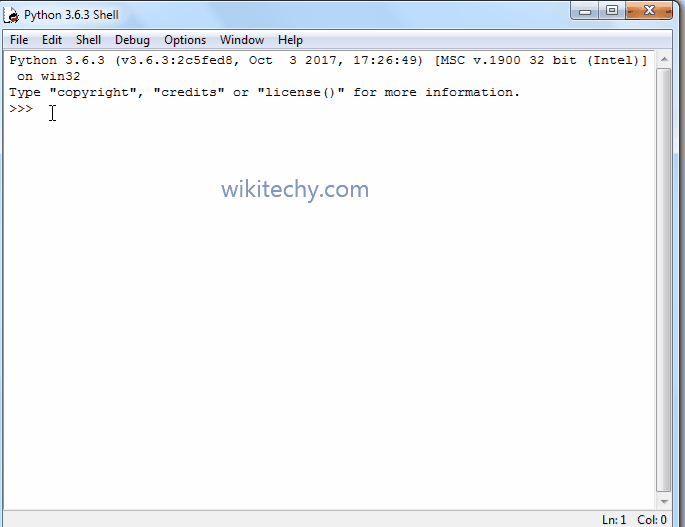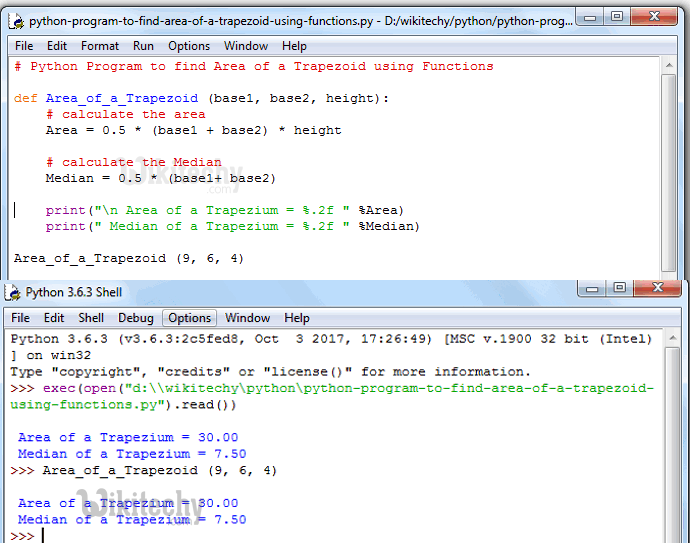# python tutorial - Python Program to find Area of a Trapezoid - learn python - python programming

• To write Python Program to find Area of a Trapezoid and Median of a Trapezoid with example. Before we step into practical example, Let see the definitions and formulas behind the Median and Area of a TrapezoidLearn Python - Python tutorial - Python Program to find Area of a Trapezoid - Python examples - Python programs

## Area of a Trapezoid

• If we know the height and two base lengths then we can calculate the Area of a Trapezoid using the below formula:
• Where a and b are the two bases and h is the height of the Trapezoid
• We can calculate the median of a Trapezoid using the following formula:
• If we know the Median and height then we can calculate the Area of a Trapezoid as: median * height

## Python Program to find Area of a Trapezoid

• This program allows the user to enter both sides of the Trapezoid and height.
• Using those values we will calculate the Area of a trapezoid and Median of a Trapezoid.

## Analysis

• Below statements will ask the user to enter base1, base2 and height values and it will assign the user input values to respected variables.
• Such as first value will be assigned to base1, second value to base2 and third value to height
• Next, we are calculating the Median and Area of a Trapezoid using their respective Formulas:
• Following print statements will help us to print the Median and Area of a Trapezoid
• Let us see the Output of a Program

## OutputLearn Python - Python tutorial - Python Program to find Area of a Trapezoid - Python examples - Python programs

• From the above screenshot you can observe that, Values that we entered are base1 = 6, base2 = 9 and height = 4

Area of a Trapezoid = 0.5 * (base1 + base2) * height;
Area of a Trapezoid = 0.5 * (6 + 9) * 4;
Area of a Trapezoid = 0.5 * 15 * 4;
Area of a Trapezoid = 30

Median of a Trapezoid = 0.5 * (base1+ base2);
Median of a Trapezoid = 0.5 * (6 + 9)
Median of a Trapezoid = 0.5 * 15
Median of a Trapezoid = 7.5

## Python Program to find Area of a Trapezoid using functions

• This program allows the user to enter the base1, base2 and height of a Trapezoid.
• We will pass those values to the function arguments to calculate the area of a Trapeziod.

## Analysis

• First, We defined the function with three arguments using def keyword.
• It means, User will enter the base1, base2 and height of a Trapezoid.
• Next, We are Calculating the Median and Area of a Trapezoid as we described in our first example.

## OutputLearn Python - Python tutorial - Python Program to find Area of a Trapezoid using functions - Python examples - Python programs

NOTE: We can call the function with arguments in .py file directly or else we can call it from the python shell. Please don’t forget the function arguments